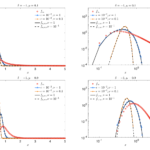# Control of tumour growth distributions through kinetic methodsL. Preziosi, G. Toscani, M. Zanella

Journal of Theoretical Biology, 514: 110579, 2021. (Preprint arXiv)

The mathematical modeling of tumor growth has a long history, and has been mathematically formulated in several different ways. Here we tackle the problem in the case of a continuous distribution using mathematical tools from statistical physics. To this extent, we introduce a novel kinetic model of growth which highlights the role of microscopic transitions in determining a variety of equilibrium distributions. At variance with other approaches, the mesoscopic description in terms of elementary interactions allows to design precise microscopic feedback control therapies, able to influence the natural tumor growth and to mitigate the risk factors involved in big sized tumors. We further show that under a suitable scaling both the free and controlled growth models correspond to Fokker–Planck type equations for the growth distribution with variable coefficients of diffusion and drift, whose steady solutions in the free case are given by a class of generalized Gamma densities which can be characterized by fat tails. In this scaling the feedback control produces an explicit modification of the drift operator, which is shown to strongly modify the emerging distribution for the tumor size. In particular, the size distributions in presence of therapies manifest slim tails in all growth models, which corresponds to a marked mitigation of the risk factors. Numerical results confirming the theoretical analysis are also presented.